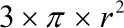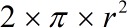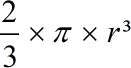Request a Tool

Volume of a Hemisphere Calculator

The volume of a cylinder with hemispherical ends is calculated by entering the known values for radius and length.

Surface Area
0

Formula• π = a constant value 3.1415
• r = Radius of the hemisphere

Defination / Uses

On one side of a great circle, a hemisphere is half of a sphere. Hemisphere is defined as the precise half of a sphere in geometry. The Northern Hemisphere and the Southern Hemisphere are two halves of the globe.

Output

Curved Surface Area
0

Formula• π = a constant value 3.1415
• r = Radius of the hemisphere

Defination / Uses

On one side of a great circle, a hemisphere is half of a sphere. Hemisphere is defined as the precise half of a sphere in geometry. The Northern Hemisphere and the Southern Hemisphere are two halves of the globe.

Volume
0

Formula• π = a constant value 3.1415
• r = Radius of the hemisphere

Defination / Uses

On one side of a great circle, a hemisphere is half of a sphere. Hemisphere is defined as the precise half of a sphere in geometry. The Northern Hemisphere and the Southern Hemisphere are two halves of the globe.

How to use hemisphere calcultor?

There are only two basic steps.

• First select the option from dropdown. What you need to calculate.
• Then add the other values in their corresponding fields.

Thats it! You will get your answer in just a second. use upper given formula in case of manual calculation

Our hemisphere surface area calculator is a useful tool for calculating various hemisphere surface areas. As shown in the diagram below, hemispheres are made by dividing a spherical into two equal half. A hemisphere, unlike a whole sphere, includes two types of surface areas: the base (which is a circle) and the cap (which is a sphere). The entire volume of two hemispheres equals the volume of one full sphere, which is an interesting fact. Surface areas, on the other hand, are not the same. The combined area of two hemispheres exceeds that of a sphere. The rationale is straightforward: hemispheres have a larger base area.